# Absolute value + complex numbers - math problems

#### Number of problems found: 6

• ABS CNCalculate the absolute value of complex number -15-29i.
• The modulus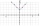Find the modulus of the complex number 2 + 5i
• Goniometric form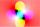Determine the goniometric form of a complex number ?.
• Log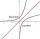Calculate value of expression log |3 +7i +5i2| .
• Modulus and argument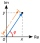Find the mod z and argument z if z=i
• Moivre 2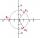Find the cube roots of 125(cos 288° + i sin 288°).

We apologize, but in this category are not a lot of examples.
Do you have an interesting mathematical word problem that you can't solve it? Submit a math problem, and we can try to solve it.

We will send a solution to your e-mail address. Solved examples are also published here. Please enter the e-mail correctly and check whether you don't have a full mailbox.

Please do not submit problems from current active competitions such as Mathematical Olympiad, correspondence seminars etc...
Try our complex numbers calculator. Absolute value - math problems. Complex numbers - math problems.